# Adding Straight Lines to a Plot in R Programming – abline() Function

• Last Updated : 14 Jul, 2020

`abline()` function in R Language is used to add one or more straight lines to a graph. The `abline()` function can be used to add vertical, horizontal or regression lines to plot.

Syntax:
abline(a=NULL, b=NULL, h=NULL, v=NULL, …)

Parameters:
a, b: It specifies the intercept and the slope of the line
h: specifies y-value for horizontal line(s)
v: specifies x-value(s) for vertical line(s)

Returns: a straight line in the plot

Example 1: To add a vertical line to the plot

 `# add line to square plot  ` `# first example : Add one line ` `plot``(cars) ` `abline``(v = 16, col = ``"darkgreen"``) ` ` `  `# second example : add 2 lines  ` `# addline to square plot  ` `# change line colors, sizes and types ` `plot``(cars) ` `abline``(v = ``c``(16, 22), col = ``c``(``"darkgreen"``, ``"blue"``), ` `                      ``lty = ``c``(1, 2), lwd = ``c``(1, 3)) ` ` `  `# third example ` `set.seed``(1200); mydata<-``rnorm``(180) ` `hist``(mydata, col=``"darkgreen"``) ` ` `  `# lwd=line width, lty =linetype  ` `abline``(v = ``mean``(mydata), col = ``"blue"``, lwd = 4, lty = 4)  `

Output: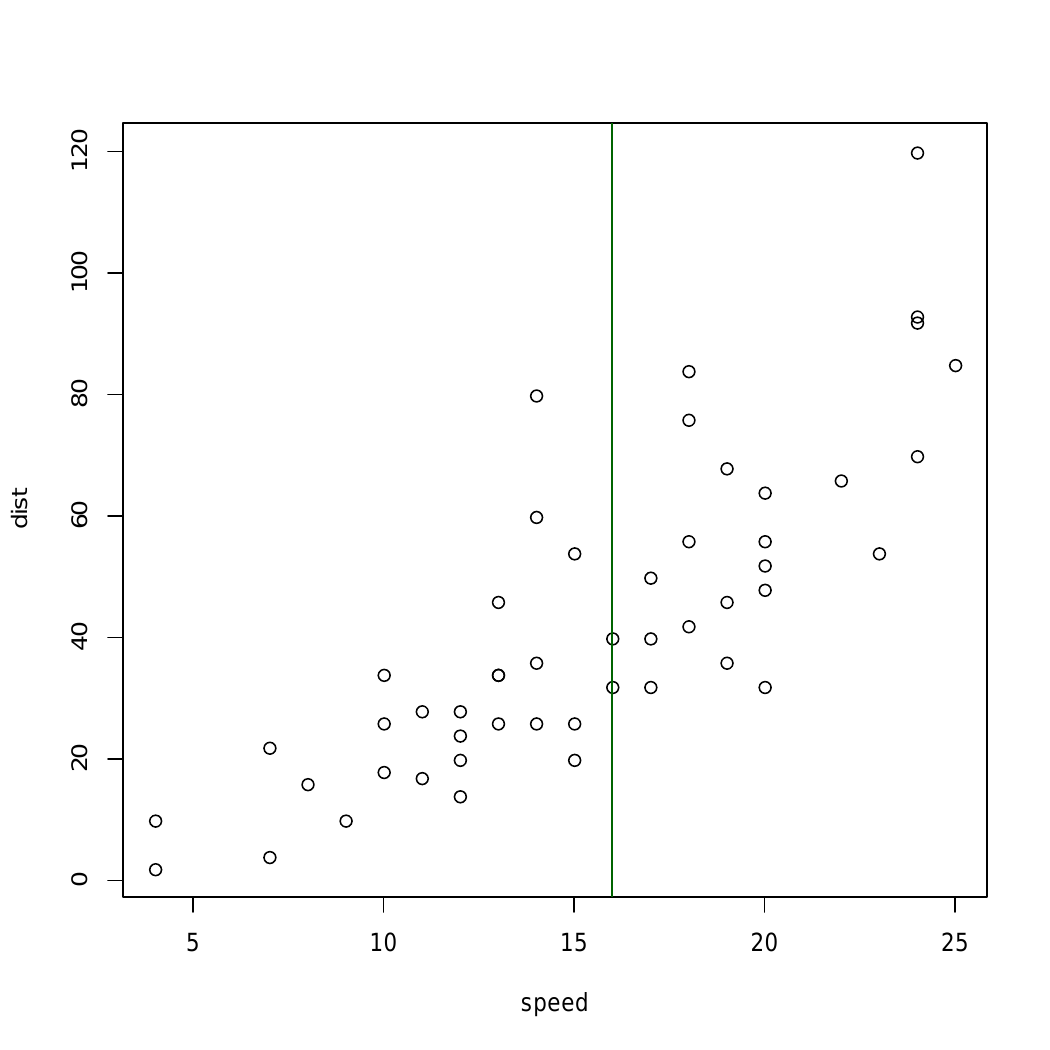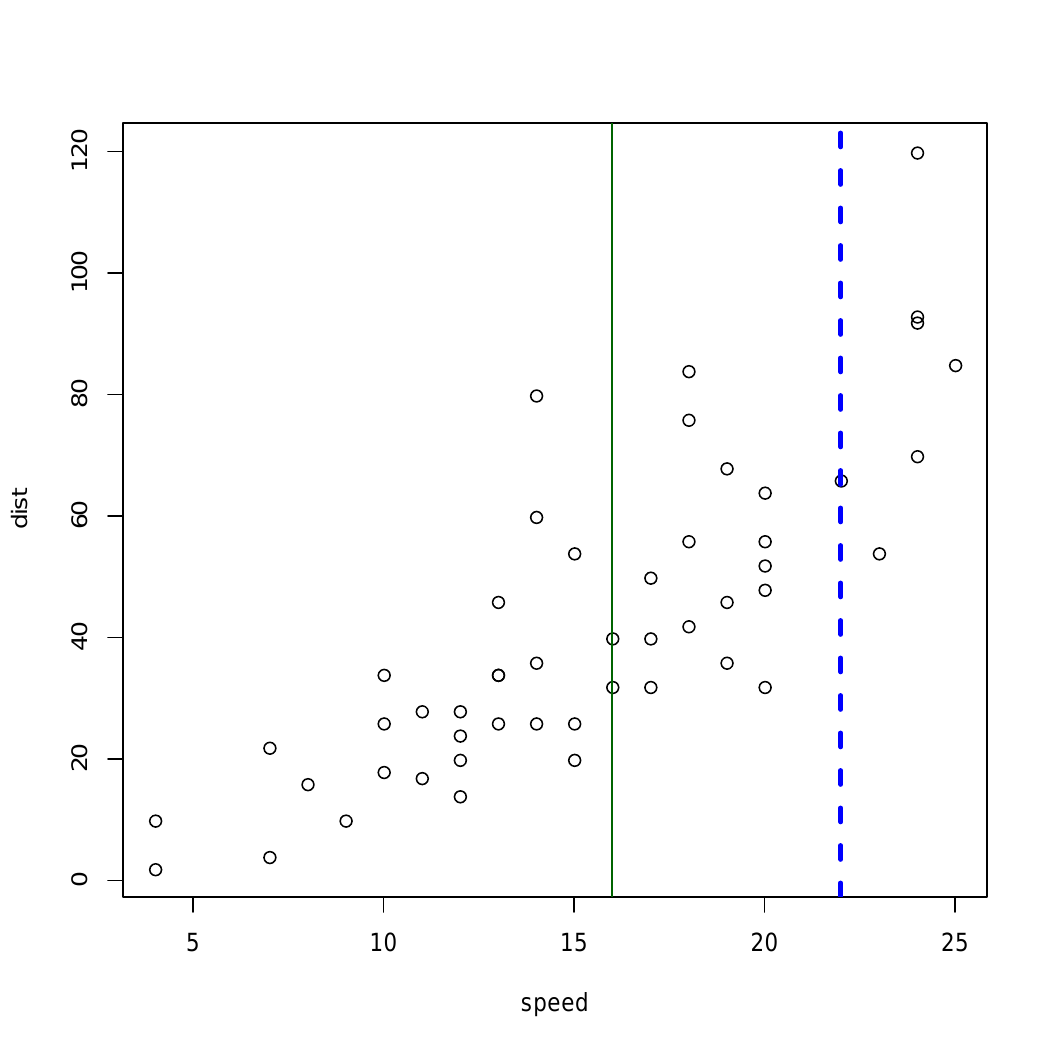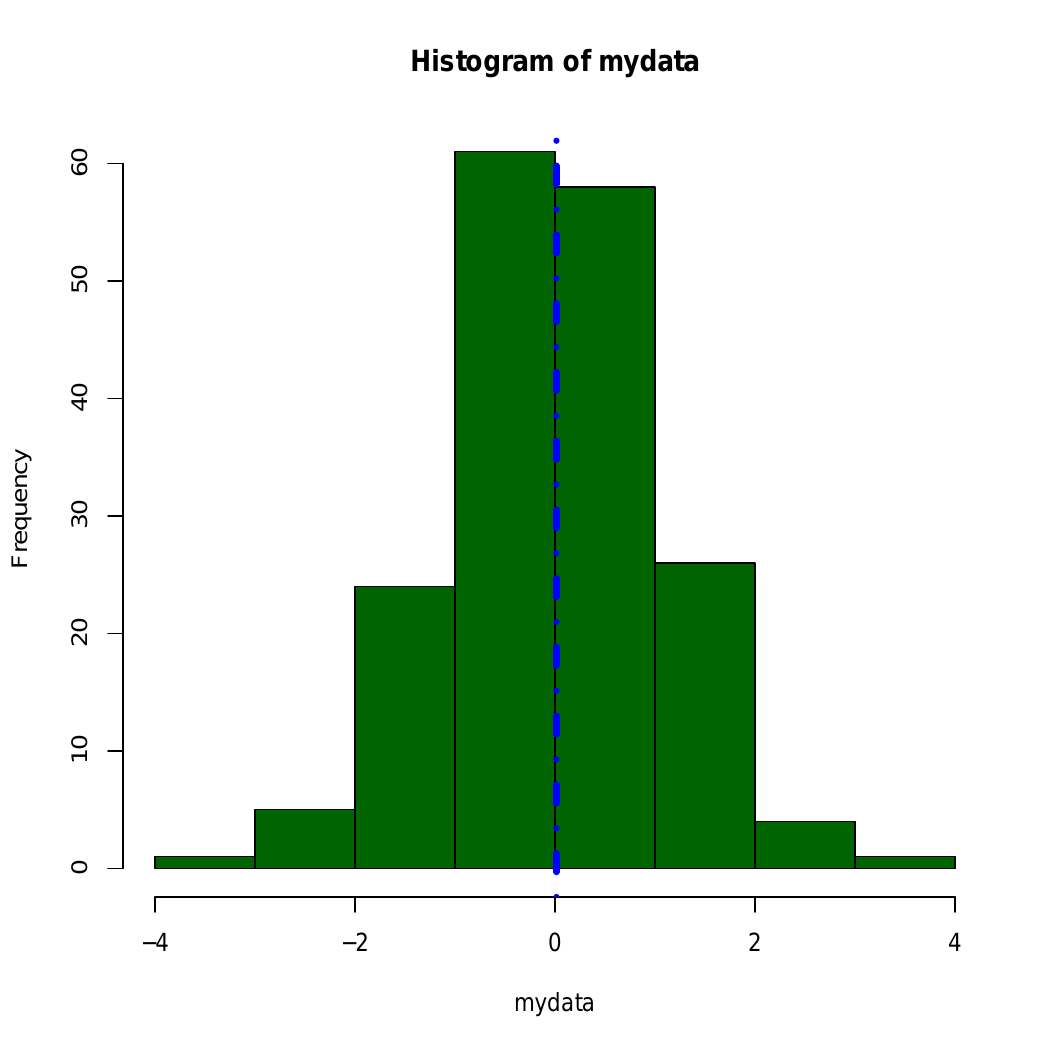Here, in above example straight line is added using abline() to different graphical plots

Example 2: To add a horizontal line

 `# R program to add a horizontal line ` `# to a plot ` ` `  `# Creating a plot ` `plot``(cars) ` ` `  `# Calling abline() function ` `abline``(h = 60, col = ``"darkgreen"``) `

Output: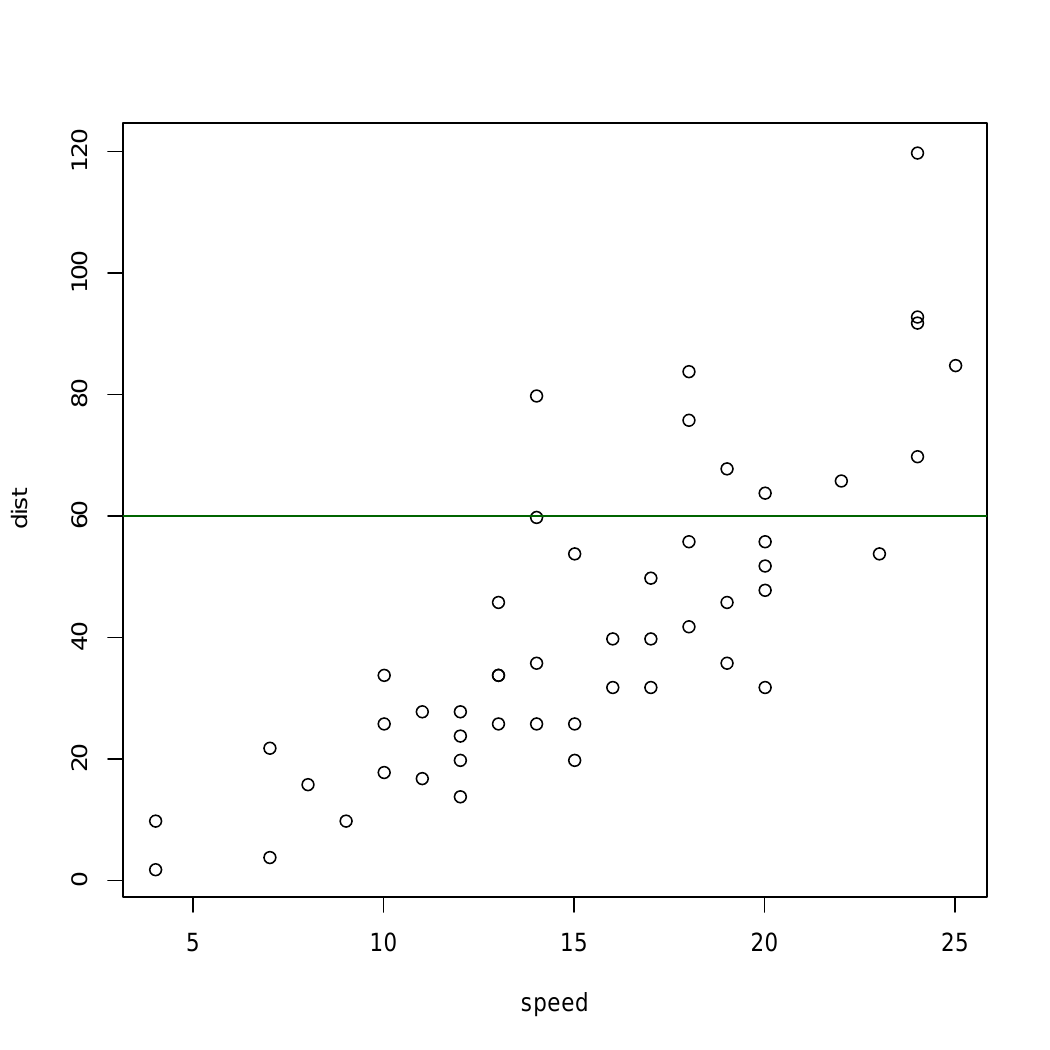In above example `abline()` Function draws an horizontal line on the current plot at the specified ‘x’ coordinates.

Example 3: To add a regression line

 `par``(mgp = ``c``(2, 1, 0), mar = ``c``(3, 3, 1, 1)) ` ` `  `# Fit regression line ` `require``(stats) ` `reg<-``lm``(dist ~ speed, data = cars) ` `coeff = ``coefficients``(reg) ` ` `  `# equation of the line :  ` `eq = ``paste0``(``"y = "``, ``round``(coeff, 1), ``"*x "``,  ` `                    ``round``(coeff, 1)) ` ` `  `# plot ` `plot``(cars, main = eq) ` `abline``(reg, col = ``"darkgreen"``) `

Output: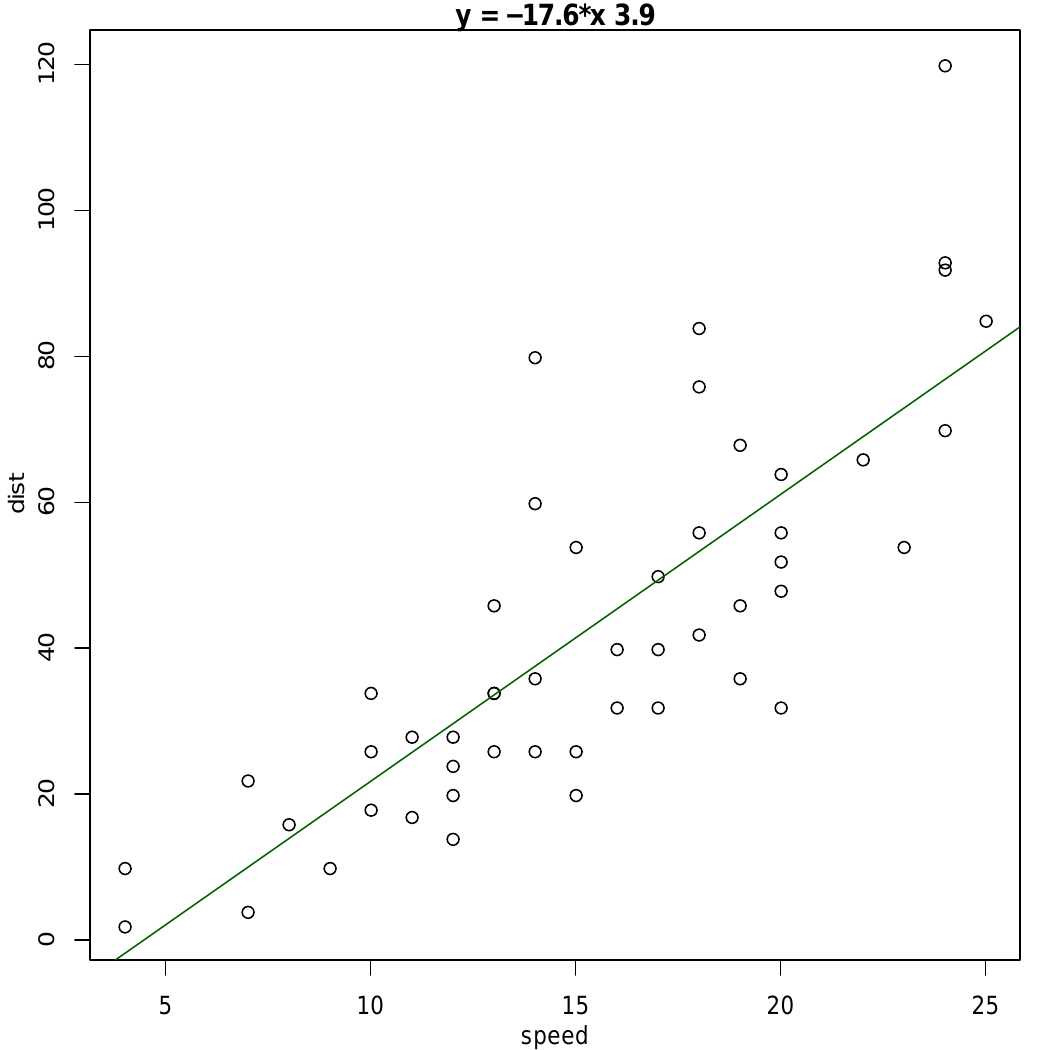In the above example, straight-line is added using the line equation and `abline()` function and plot relation between speed and distance.

My Personal Notes arrow_drop_up
Recommended Articles
Page :